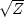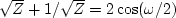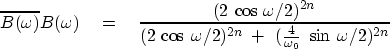Next: Examples of Butterworth filters Up: A BUTTERWORTH-FILTER COOKBOOK Previous: A BUTTERWORTH-FILTER COOKBOOK

## Butterworth-filter finding program

To express equation (23) in the Fourier domain, multiply every parenthesized factor byand recall that.Thus,(25)
An analogous equation holds for high-pass filters. Subroutine butter()does both equations. First, the denominator of equation (25) is set up as a spectrum and factored. The numerator could be found in the same way, but the result is already apparent from the numerator of (23), i.e., we need the coefficients of (1+Z)n. In subroutine butter() they are simply obtained by Fourier transformation. The occurrence of a tangent in the program arises from equation ().
# Find the numerator and denominator Z-transforms of the Butterworth filter.
#   hilo={1.,-1.} for {low,high}-pass filter
#   cutoff in Nyquist units, i.e. cutoff=1 for (1,-1,1,-1...)
#
subroutine butter( hilo, cutoff, npoly, num, den)
integer npoly, nn, nw, i
real hilo, cutoff, num(npoly), den(npoly), arg, tancut, pi
complex cx(2048)
pi = 3.14159265;        nw=2048;        nn = npoly - 1
tancut = 2. * tan( cutoff*pi/2. )
do i= 1, nw {
arg = (2. * pi * (i-1.) / nw) / 2.
if( hilo > 0. )                                 # low-pass filter
cx(i) = (2.*cos(arg)             ) **(2*nn) +
(2.*sin(arg) * 2./tancut ) **(2*nn)
else                                            # high-pass filter
cx(i) = (2.*sin(arg)             ) **(2*nn) +
(2.*cos(arg) * tancut/2. ) **(2*nn)
}
call kolmogoroff( nw, cx)       # spectral factorization
do i= 1, npoly
den(i) = cx(i)
do i= 1, nw                     # numerator
cx(i) = (1. + hilo * cexp( cmplx(0., 2.*pi*(i-1.)/nw))) ** nn
call ftu( -1., nw, cx)
do i= 1, npoly
num(i) = cx(i)
return; endNext: Examples of Butterworth filters Up: A BUTTERWORTH-FILTER COOKBOOK Previous: A BUTTERWORTH-FILTER COOKBOOK
Stanford Exploration Project
10/21/1998